Home
Hostname: page-component-79b67bcb76-b5nxq Total loading time: 0.219 Render date: 2021-05-15T12:33:15.332Z Has data issue: true Feature Flags: { "shouldUseShareProductTool": true, "shouldUseHypothesis": true, "isUnsiloEnabled": true, "metricsAbstractViews": false, "figures": false, "newCiteModal": false, "newCitedByModal": true, "newEcommerce": true }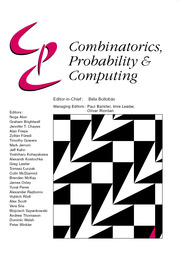Combinatorics, Probability and Computing

# Dirac’s theorem for random regular graphs

Published online by Cambridge University Press:  28 August 2020

Corresponding

## Abstract

We prove a ‘resilience’ version of Dirac’s theorem in the setting of random regular graphs. More precisely, we show that whenever d is sufficiently large compared to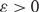$\epsilon > 0$ , a.a.s. the following holds. Let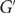$G'$ be any subgraph of the random n-vertex d-regular graph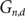$G_{n,d}$ with minimum degree at least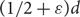$$(1/2 + \epsilon )d$$ . Then$G'$ is Hamiltonian.

This proves a conjecture of Ben-Shimon, Krivelevich and Sudakov. Our result is best possible: firstly the condition that d is large cannot be omitted, and secondly the minimum degree bound cannot be improved.

## MSC classification

Type
Paper
Information
Combinatorics, Probability and Computing , January 2021 , pp. 17 - 36

## Access options

Get access to the full version of this content by using one of the access options below.

## Footnotes

This project has received partial funding from the European Research Council (ERC) under the EuropeanUnion’sHorizon 2020 research and innovation programme (grant agreement 786198, D. Kühn and D. Osthus). The research leading to these results was also partially supported by the EPSRC, grants EP/N019504/1 (A. Girão and D. Kühn) and EP/S00100X/1 (D. Osthus), as well as the Royal Society and theWolfson Foundation (D. Kühn).

## References

Ajtai, M., Komlós, J. and Szemerédi, E. (1985) First occurrence of Hamilton cycles in random graphs. In Cycles in Graphs (Burnaby, BC, 1982), Vol. 115 of North-Holland Mathematics Studies, pp. 173178. North-Holland.Google Scholar
Allen, P., Böttcher, J., Ehrenmüller, J. and Taraz, A. (2020) The bandwidth theorem in sparse graphs. Adv. Combin. 2020. doi: 10.19086/aic.12849 CrossRefGoogle Scholar
Alon, N. and Spencer, J. H. (2016) The Probabilistic Method, Wiley Series in Discrete Mathematics and Optimization. Wiley.Google Scholar
Azuma, K. (1967) Weighted sums of certain dependent random variables. Tôhoku Math. J. (2) 19 357367.CrossRefGoogle Scholar
Balogh, J., Csaba, B. and Samotij, W. (2011) Local resilience of almost spanning trees in random graphs. Random Struct. Algorithms 38 121139.Google Scholar
Balogh, J., Lee, C. and Samotij, W. (2012) Corrádi and Hajnal’s theorem for sparse random graphs. Combin. Probab. Comput. 21 2355.CrossRefGoogle Scholar
Ben-Shimon, S., Krivelevich, M. and Sudakov, B. (2011) Local resilience and Hamiltonicity maker-breaker games in random regular graphs. Combin. Probab. Comput. 20 173211.CrossRefGoogle Scholar
Ben-Shimon, S., Krivelevich, M. and Sudakov, B. (2011) On the resilience of Hamiltonicity and optimal packing of Hamilton cycles in random graphs. SIAM J. Discrete Math. 25 11761193.Google Scholar
Bollobás, B. (1980) A probabilistic proof of an asymptotic formula for the number of labelled regular graphs. European J. Combin. 1 311316.Google Scholar
Bollobás, B. (1984) The evolution of sparse graphs. In Graph Theory and Combinatorics (Cambridge, 1983), pp. 3557. Academic Press.Google Scholar
Condon, P., Espuny Díaz, A., Girão, A., Kühn, D. and Osthus, D. (2019) Dirac’s theorem for random regular graphs. arXiv:1903.05052Google Scholar
Condon, P., Espuny Daz, A., Kim, J., Kühn, D. and Osthus, D. (2019) Resilient degree sequences with respect to Hamilton cycles and matchings in random graphs. Electron. J. Combin. 26 4.54.Google Scholar
Cook, N., Goldstein, L. and Johnson, T. (2018) Size biased couplings and the spectral gap for random regular graphs. Ann. Probab. 46 72125.CrossRefGoogle Scholar
Cooper, C., Frieze, A. and Reed, B. (2002) Random regular graphs of non-constant degree: connectivity and Hamiltonicity. Combin. Probab. Comput. 11 249261.CrossRefGoogle Scholar
Ferber, A., Nenadov, R., Noever, A., Peter, U. and Škorić, N. (2017) Robust Hamiltonicity of random directed graphs. J. Combin. Theory Ser. B 126 123.CrossRefGoogle Scholar
Frieze, A. (2019) Hamilton cycles in random graphs: a bibliography. arXiv:1901.07139Google Scholar
Frieze, A. and Krivelevich, M. (2008) On two Hamilton cycle problems in random graphs. Israel J. Math. 166 221234.CrossRefGoogle Scholar
Hefetz, D., Steger, A. and Sudakov, B. (2016) Random directed graphs are robustly Hamiltonian. Random Struct. Algorithms 49 345362.CrossRefGoogle Scholar
Hoeffding, W. (1963) Probability inequalities for sums of bounded random variables. J. Amer. Statist. Assoc. 58 1330.CrossRefGoogle Scholar
Huang, H., Lee, C. and Sudakov, B. (2012) Bandwidth theorem for random graphs. J. Combin. Theory Ser. B 102 1437.CrossRefGoogle Scholar
Janson, S., Łuczak, T. and Ruciński, A. (2000) Random Graphs, Wiley-Interscience Series in Discrete Mathematics and Optimization. Wiley-Interscience.CrossRefGoogle Scholar
Kim, J. H. and Vu, V. H. (2004) Sandwiching random graphs: universality between random graph models. Adv. Math. 188 444469.CrossRefGoogle Scholar
Komlós, J. and Szemerédi, E. (1983) Limit distribution for the existence of Hamiltonian cycles in a random graph. Discrete Math. 43 5563.CrossRefGoogle Scholar
Krivelevich, M. (2016) Long paths and Hamiltonicity in random graphs. In Random Graphs, Geometry and Asymptotic Structure (Fountoulakis, N. and Hefetz, D., eds), Vol. 84 of London Mathematical Society Student Texts, pp. 427. Cambridge University Press.Google Scholar
Krivelevich, M., Lee, C. and Sudakov, B. (2010) Resilient pancyclicity of random and pseudorandom graphs. SIAM J. Discrete Math. 24 116.Google Scholar
Krivelevich, M., Sudakov, B., Vu, V. H. and Wormald, N. C. (2001) Random regular graphs of high degree. Random Struct. Algorithms 18 346363.CrossRefGoogle Scholar
Lee, C. and Sudakov, B. (2012) Dirac’s theorem for random graphs. Random Struct. Algorithms 41 293305.Google Scholar
McKay, B. D. (1987) Independent sets in regular graphs of high girth. Ars Combin. 23 179185.Google Scholar
McKay, B. D. and Wormald, N. C. (1990) Uniform generation of random regular graphs of moderate degree. J. Algorithms 11 5267.Google Scholar
Montgomery, R. (2019) Hamiltonicity in random graphs is born resilient. J. Combin. Theory Ser. B 139 316341.CrossRefGoogle Scholar
Montgomery, R. (2020) Hamiltonicity in random directed graphs is born resilient. Combin. Probab. Comput. doi: 10.1017/S0963548320000140.CrossRefGoogle Scholar
Nenadov, R., Steger, A. and Trujić, M. (2019) Resilience of perfect matchings and Hamiltonicity in random graph processes. Random Struct. Algorithms 54 797819.Google Scholar
Robinson, R. W. and Wormald, N. C. (1992) Almost all cubic graphs are Hamiltonian. Random Struct. Algorithms 3 117125.CrossRefGoogle Scholar
Robinson, R. W. and Wormald, N. C. (1994) Almost all regular graphs are Hamiltonian. Random Struct. Algorithms 5 363374.CrossRefGoogle Scholar
Škorić, N., Steger, A. and Trujić, M. (2018) Local resilience of an almost spanning k-cycle in random graphs. Random Struct. Algorithms 53 728751.CrossRefGoogle Scholar
Sudakov, B. and Vu, V. H. (2008) Local resilience of graphs. Random Struct. Algorithms 33 409433.CrossRefGoogle Scholar
Tikhomirov, K. and Youssef, P. (2019) The spectral gap of dense random regular graphs. Ann. Probab. 47 362419.Google Scholar
Wormald, N. C. (1999) Models of random regular graphs. In Surveys in Combinatorics (Canterbury, 1999), Vol. 267 of London Mathematical Society Lecture Note Series, pp. 239298. Cambridge University Press.Google Scholar

# Send article to Kindle

Note you can select to send to either the @free.kindle.com or @kindle.com variations. ‘@free.kindle.com’ emails are free but can only be sent to your device when it is connected to wi-fi. ‘@kindle.com’ emails can be delivered even when you are not connected to wi-fi, but note that service fees apply.

Find out more about the Kindle Personal Document Service.

Dirac’s theorem for random regular graphs
Available formats
×

# Send article to Dropbox

To send this article to your Dropbox account, please select one or more formats and confirm that you agree to abide by our usage policies. If this is the first time you use this feature, you will be asked to authorise Cambridge Core to connect with your <service> account. Find out more about sending content to Dropbox.

Dirac’s theorem for random regular graphs
Available formats
×

# Send article to Google Drive

To send this article to your Google Drive account, please select one or more formats and confirm that you agree to abide by our usage policies. If this is the first time you use this feature, you will be asked to authorise Cambridge Core to connect with your <service> account. Find out more about sending content to Google Drive.

Dirac’s theorem for random regular graphs
Available formats
×
×